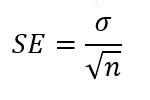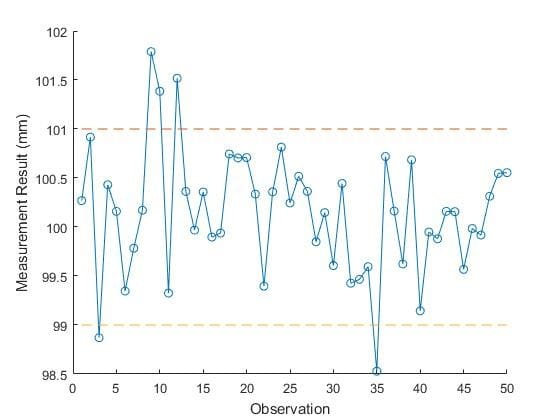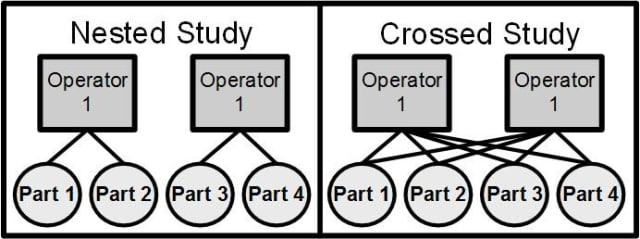Gage Studies and Gage R&R
Jody Muelaner posted on December 18, 2017 |
Understanding bias, variation, repeatability and reproducibility in manufacturing.If you’ve ever been involved with an industrial measurement process, chances are you’ve come across Gage studies and in particular Gage Repeatability and Reproducibility (Gage R&R).

These tests are used to determine the accuracy of measurements. In simple terms, measurements are repeated and the results are used to calculate variation and bias. If you’ve read my previous articles on uncertainty of measurement, you’ll probably notice that the language used here is a little different. This is because Gage R&R studies are part of the Measurement Systems Analysis (MSA) approach.

In MSA, accuracy is defined as the combination of trueness (bias) and precision (variation), while uncertainty is evaluated by combining Type A estimates (experimental variation data) with Type B estimates (other estimates of uncertainties). MSA only uses experimental data, which prevents false assumptions but may also mean that some significant uncertainties may be ignored because they cannot practically be experimentally measured.

I’ll go into the pros and cons of these approaches, and the relationship between them, in a future article. In this article, I explain how gage studies work and what they are useful for.

The two main types of gage study are the Type 1 Gage Study and the Gage Repeatability and Reproducibility (Gage R&R) Study. A Type 1 Gage Study is a relatively simple and quick check which should be done first to determine the bias and repeatability of a measurement system. A Gage R&R Study is a more in-depth study which can be used to identify variation in both the measurement system and the manufacturing process, and to identify individual sources of this variation.

## Type 1 Gage Study

A Type 1 Gage Study is a relatively simple and quick check in which a calibrated reference part is measured many times within a short period of time to determine the bias and repeatability of a measurement system. This study should be done before a Gage R&R study. The recommended number of repeated measurements is over 50.

Bias is calculated by first finding the mean of the repeated measurements and then subtracting the reference value. The reference value is obtained by measuring the reference part using a significantly more accurate measurement system. Ideally, this reference measurement should have an uncertainty no greater than 1/10th of the measurement being studied. However, this may not be achievable in practice, which is why calibration uncertainty is explicitly considered in an uncertainty evaluation approach.

Repeatability or precision is expressed as a standard deviation, found by simply taking the standard deviation of all of the repeated measurements. A test is then applied to check whether the bias is significant. If the bias is small in comparison to the random variation in the measurements, then we can imagine that if we repeated the study we might see an entirely different bias, perhaps even changes from positive to negative.

In such a case, we could say that there is no bias and that any observed bias is simply the result of the random variation. This random variation in the observed bias is known in statistics as the Standard Error in the Mean. This can be found by taking the standard deviation for the repeated measurements (the repeatability of precision) and dividing it by the square root of the number of measurements (n). In equation form:The likelihood that the observed bias is purely a result of the random variation is related to the size of the bias in relation to the Standard Error. Assuming a normal distribution, if the bias is more than two Standard Errors then the chance that it is purely a result of the random variation is less than 5 percent and it is deemed to be significant. Whether the results can be deemed to be normally distributed and more importantly whether 5 percent is the right level of chance to apply are subjective judgement calls.

A run chart and a histogram are often used to evaluate whether results are random and normally distributed. In a run chart, each measurement is plotted in order, allowing trends such as drift and oscillation to be clearly identified. If there are no evident trends, then it’s reasonable to assume that the variation is random. Run charts are really useful tools which can be very easily applied. They really help you see what is going on with the variation in a system.A run chart is a really useful way of understanding variation, identifying any trends and determining whether the variation is random. (Image courtesy of the author.)
A histogram is a graphical representation of a probability distribution. If the measurement variation is normally distributed, then most of the values will be close to the mean with a small number of values in the ‘tails’ on either side. If the variation in uniformly distributed then there will be an even number of values spread out over the range.

It is important to remember that unless you are dealing with extremely large samples sizes (thousands to millions), what you see will be a very rough approximation to the expected distribution.A Histogram for 50 randomly generated samples from a normal distribution, note that it only very roughly approximates the bell shaped curve of the underlying distribution. (Image courtesy of the author.)

### Gage Capability

We often want to know if a measurement is capable of determining whether or not a part is within specification. Performing a gage study can yield an answer to this question.

In the extreme worst case, the measurement variation is larger than the product tolerance. In such an event, even if every product is produced to exactly the nominal dimensions, the measurement results would show the parts as being randomly in or out of tolerance. Conversely, products which are well outside of tolerance could be shown to be perfect.

In the extreme best case, there would be no bias or variation in the measurement system and we could be 100 percent confident in the measurement results’ ability to distinguish between conforming and non-conforming products.

Clearly, we will usually be somewhere between these extreme cases and must decide if the measurement system is sufficiently capable.

Fundamentally, we are concerned with the ratio between the accuracy of the measurement system and the product’s tolerance. If we use the ratio between the precision and the tolerance, then this gives the potential capability assuming corrections are made for bias. Typically, a ratio of 10 percent to 20 percent is regarded as acceptable.

It’s also possible to estimate the probability of making a false decision using some basic statistics (since the inverse of this ratio is effectively a z-score) – I’ll explain more about that in a future post.

Gage capability is also commonly expressed as Cg and Cgk. Cg refers to the ratio between precision and tolerance (the potential capability) while Cgk denotes the ratio between the accuracy and the tolerance (the actual capability). I would, however, advise against using Cg and Cgk.

The reason for this is that they introduce some additional arbitrary factors into the ratio which obscure its true meaning and prevent further statistical analysis. For example, Cg is given by:In this equation Tol is the process tolerance and σ is the standard deviation in the measurements (the precision). Therefore, without the additional factors, this would give the sigma level (z-score or inverse of the ratio used above). The additional factors are K, the percentage of the tolerance (typically 20 percent), 100 to convert K from a percentage to a proportion and L: the number of standard deviations thought to represent the actual or target process spread.

### Gage R&R Study

A Gage Repeatability and Reproducibility (a.k.a. Gage R&R) study is normally carried out as the next stage after completing a Type 1 Gage Study. Gage R&R provides a deeper understanding of the variation in the process and measurement system, typically allowing individual sources of variation to be quantified.

There are three types of gage R&R study:

1. Crossed
2. Nested
3. Expanded

A crossed study is the most common type of Gage R&R study, most often used where we are able to instruct each operator to measure each part a fixed number of times. These must be continuous measurements, which means that the result is a number on a scale, as opposed to an attribute gage which gives a Go/No-Go result. Generally, we will have 10 parts and 3 operators who each measure each part 3 times for a total of 90 measurements.

A crossed study is sometimes used for destructive tests in which each physical part can only actually be measured once ,since they are destroyed or changed by the measurement or test. Provided we can obtain samples which are so similar that we can consider them to be the same part, we can use these in a crossed study and simply label the results as if the measurements came from the same part. Care should, of course, be taken to ensure that this homogeneity assumption is valid.

In some cases of carrying out a gage study for a destructive test, it’s possible to obtain nine parts which are similar enough that we can consider them to be the same part. If we can obtain six parts, then three operators can measure each part twice and this is still acceptable for a crossed study.

However, if we are only able to obtain two similar parts at a time, then each operator must measure different parts. In that case, we need to treat the data very differently and use a nested study, where each operator measures a different group of parts. Consequently, the parts are nested within the operator, rather than crossed as shown in the diagram.(Image courtesy of the author.)
The final type of study, the expanded study, is really a bit of a catch-all category for any non-standard study. This may mean including additional factors, specifying whether factors are fixed or random and/or analysing data for unbalanced studies.

Conventional wisdom says you should only consider two factors in a gage R&R study: parts and operators. In reality, other factors may also have an effect or even be more significant than the traditional pair of part and operator.

For example, environmental factors such as temperature and humidity may be important for laser based measurements and ambient light may be significant for non-contact scanning. The speed of a line may influence the operator’s ability to measure correctly, and so line speed can be another important factor.

In a crossed study, we assume that parts and operators are randomly selected and then carry out our analysis for these random factors. If you were to deliberately select parts covering the full range of variation and operators covering the full range of experience, then these are said to be fixed factors. The analysis should be carried out a little differently in this case. In an expanded study it is possible to specify whether each factor is fixed or random.

A crossed study requires that all of the parts are measured the same number of times by each operator. This is known as a balanced design. However, in a production environment there may be cases where it isn’t practical for all the operators to measure all the parts an equal number of times— some of the measurement data may even have been lost.

If this is the case, you have an unbalanced design. An expanded study can adapt the analysis to cope with these unbalanced designs.

Since a crossed study is by far the most common, let’s look at it in a bit more detail.

Remember, typically this will involve 3 operators each measuring the same 10 parts 3 times, for a total of 90 measurements. There should be at least 10 parts chosen to represent the actual or expected part-to-part variation in the process. The parts should be taken at different times, on different shifts and from different machines or lines. Each measurement result is recorded along with the part which was measured and the operator who measured it.

There a number of ways of analysing the data, some of which can be more easily performed by hand. The most accurate method is using Analysis of Variance (ANOVA). This can be easily carried out using software such as Minitab and it’s even possible to do a Gage R&R ANOVA in Excel, if you know how. So, I would always recommend using this analysis type.

ANOVA works similarly to the way in which you calculate standard deviation, but it also allows you to identify the different factors that contribute to variation. Standard deviation is essentially the average distance of the individual values from their mean value. To find this, you must first find the mean, then the difference between the mean and each value. Each of these differences is squared to remove its sign, the average of the squared differences is found and then the square root of this average is taken to remove the effect of squaring the differences previously.

ANOVA works in a very similar way, but for each measurement there is a set of measurements which share the same part and a different set of measurements which share the same operator. Each measurement, therefore, has two means associated with it: the ‘mean by part’ which is the mean of the set of measurements which share the same part; and the ‘mean by operator’ which is the mean of the set of measurements which share the same operator. It is therefore possible to find differences from these means, which give an indication of the variation due to these factors.

In this article, I have given a general overview of the types of gage study, what they are used for and how they work. Watch out for coming articles in which I will expand on these topics in greater detail and also examine the relationship between the gage studies and uncertainty evaluation.

If you have any questions, feel free to pose them in the comments below.Dr. Jody Muelaner’s 20-year engineering career began in machine design, working on everything from medical devices to saw mills. Since 2007 he has been developing novel metrology at the University of Bath, working closely with leading aerospace companies. This research is currently focused on uncertainty modelling of production systems, bringing together elements of SPC, MSA and metrology with novel numerical methods. He also has an interest in bicycle design. Visit his website for more information.
 Recommended For You